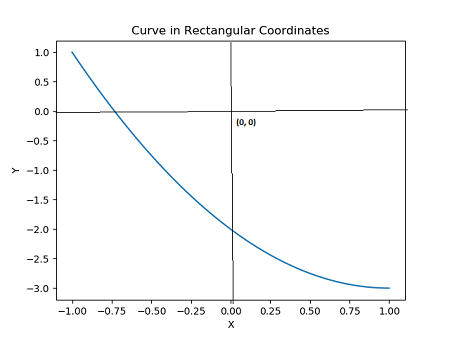# Let lambda (t) = (t - 1, t^2 - 4 t + 1) with 0 less than or equal to t less than or equal to 2...

## Question:

Let {eq}\lambda (t) = (t - 1, t^2 - 4 t + 1) {/eq} with {eq}0 \le t \le 2 {/eq}

(a) Find the rectangular equation for the curve {eq}C = Trace(\lambda).{/eq}

(b) Sketch {eq}Trace (\lambda). {/eq}

## Parametric Curve

Given a curve defined in parametric form, with parameter t, we convert it to rectangular or Cartesian coordinate form. Then using the Cartesian form we make a sketch of the graph of the curve between two given values of the parameter. The sketch is made using the PYTHON programming language package/module MATPLOTLIB.

(a) From the given parametric form we have that

From (1) get {eq}t=x+1 {/eq} and substitute in (2) to get

{eq}y=(x+1)^2-4(x+1)+1 = x^2+2x+1-4x-4+1=x^2-2x-2 \qquad (3) {/eq}

From (3) the rectangular form is {eq}y=x^2-2x-2. {/eq}

(b) Below is a sketch of the curve. The graph was sketched using the PYTHON programming language package/module MATPLOTLIB: